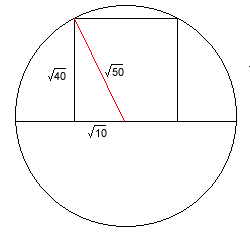#### You may also like### Some(?) of the Parts

A circle touches the lines OA, OB and AB where OA and OB are perpendicular. Show that the diameter of the circle is equal to the perimeter of the triangle### Polycircles

Show that for any triangle it is always possible to construct 3 touching circles with centres at the vertices. Is it possible to construct touching circles centred at the vertices of any polygon?### Circumspection

M is any point on the line AB. Squares of side length AM and MB are constructed and their circumcircles intersect at P (and M). Prove that the lines AD and BE produced pass through P.

# Semi-detached

##### Age 14 to 16Challenge Level

Correct solutions were recieved from Aashay of Farmington High,USA, Mary of Birchwood High School, Sana, Jenny, Chris and Rosion of Madras College, St. Andrews, Andrei of School 205 Bucharest and Chen of the Chinese High School, Singapore.If a square has an area of $40$ sq units then its side is of length $\sqrt{40} = 2 \times \sqrt{10}$.

Half the side is $\sqrt{10}$.

So radius of the circle is $\sqrt{50}$.

Applying this to the square circumscribed by the whole circle (see below)Diameter of the circle = diagonal of the square = $2 \times \sqrt 50 = \sqrt 200$

Let side of square $= x$ units. Area of the square = $x^2$

Using Pythagoras' theorem:

$x^2 + x^2 = 200$

$x^2 = 100$

Therefore the area of the square is $100$ sq units.